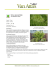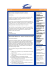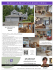# MAT 161 :: Lab Manual

## Transcription

MAT 161 :: Lab Manual
```MAT 161 :: Lab Manual
Contents
Maple Orientation
3
Limits
4
Differentiation
5
Implicit Differentiation
6
The Extreme Value Theorem
7
The Mean Value Theorem
8
Newton’s Method
9
Riemann Sums
10
Volumes of Revolution
11
3
Maple Orientation
Basic Maple Commands
1. Defining and Evaluating Functions
f := x -> x^2;
f(3);
2. Some Common Functions
f := x -> exp(x);
g := x -> sqrt(x);
3. Graphing Functions
plot(f(x),x = -3..3);
4. Solving Algebraic Equations
solve(x^2 + 2*x - 8 = 0, x);
fsolve(x^3 + x + 1 = 0, x);
5. Expanding and Factoring Polynomials
p := (x - 5)*(x + 3);
expand(p);
q := x^2 - 2*x - 15;
factor(q);
6. Evaluating Limits
f := x -> sin(x)/x;
limit(f(x), x=0);
7. Differentiating Functions
f := x -> sin(2*x);
diff(f(x), x);
D(f)(x);
8. Integrating Functions
int(x^3, x);
int(5*x, x = 0..3);
4
Limits
We can use Maple to investigate limits from numeric, graphic, and symbolic viewpoints.
restart;
f := x -> (x^2 - 4) / (x - 2);
plot(f(x),x=-5..5);
limit(f(x),x=2);
2
x −4
We observe that lim
= 4.
x→2 x − 2
Problems
Use Maple to investigate the following limits. Then sketch a plot of each function on
your own paper and write out the complete set of steps required to evaluate each limit
symbolically.
x4 − 16
lim
x→2
x−2
3
x − x2 − 5x − 3
lim
x→−1
(x + 1)2
x2 − 9
lim √
x→3
x2 + 7 − 4
1 − cos(x)
lim
x→0
x sin(x)
1.
2.
3.
4.
5
Differentiation
The Maple program provides a convenient way to compute derivatives via the limit
definition of derivative. We compute the derivative of f (x) = x21+4 with Maple by
issuing the following commands:
restart;
f := x -> 1/(x^2 + 4) ;
difference_quotient := (f(x+h)-f(x))/h ;
simplified_difference_quotient := simplify(difference_quotient);
limit_of_difference_quotient := limit(difference_quotient,h=0);
D(f);
We conclude that f 0 (x) =
−2x
.
(x2 +4)2
Problems
Use Maple as illustrated in the paradigm above to find the derivative of each of the
following functions using both the limit definition and Maple’s D operator. Then write
out on your own paper the complete set of steps required to compute each derivative.
1. f (x) = x4 − 5x
2. f (x) =
3. f (x) =
x2 +1
x−2
√
3x + 1
(
x2 sin
4. f (x) =
0
1
x
if x 6= 0
if x = 0
6
Implicit Differentiation
We use Maple to investigate the implicitly defined curve given by x3 + y 3 = 6xy. This
curve is often called a Folium of Descartes. Maple allows us to visualize the curve and
to find a slope formula for the curve through implicit differentiation.
restart;
with(plots):
implicitplot(x^3 + y^3 = 6*x*y,x=-5..5,y=-5..5);
implicitdiff(x^3 + y^3 = 6*x*y,y,x);
We observe that
dy
2y − x2
= 2
dx
y − 2x
is a slope formula for this Folium of Descartes. In particular, the slope of the curve at
the point (1, 1) is
2(1) − (1)2
dy =
= −1.
dx (x=1,y=1) (1)2 − 2(1)
Problems
Use Maple to investigate the following implicitly defined curves. Then sketch a plot
of each curve on your own paper and write out the complete set of steps required to
determine a slope formula for each curve symbolically.
1. Piriform curve: y 2 = x3 (2 − x)
2. Astroid: x2/3 + y 2/3 = 4
3. Lemniscate of Bernoulli: 2(x2 + y 2 )2 = 25(x2 − y 2 )
4. Kampyle of Eudoxus: y 2 = 5x4 − x2
7
The Extreme Value Theorem
We use Maple to find the maximum and minimum values of the continuous function
f (x) = 3x4 − 16x3 + 18x2 on the closed interval [−1, 4].
restart;
with(plots):
f := x -> 3*x^4 - 16*x^3 + 18*x^2;
plot(f(x), x = -1..4);
critical_numbers := fsolve(D(f)(x) = 0, x, x = -1..4);
seq([critical_numbers[k], f(critical_numbers[k])],
k = 1..nops([critical_numbers]));
end_points := [-1,4];
seq([end_points[k], evalf(f(end_points[k]))], k = 1..nops(end_points));
We observe that the maximum value of the function on the indicated interval is 37, and
it occurs when x = −1. The minimum value of the function on the indicated interval
is −27, and it occurs when x = 3.
Problems
Use Maple to investigate the maximum and minimum values of the following continuous
functions on the indicated closed intervals.
1. f (x) = x2 − 6x + 11,
[2, 5]
2. f (x) = x3 − 4x + 5,
[−1, 3]
3. f (x) =
x2
x
,
+1
4. f (x) = sin x,
[0, 2]
5π 0, 4
8
The Mean Value Theorem
The key idea we investigate is that if f is continuous on [a, b] and differentiable on (a, b),
then there is at least one value c ∈ (a, b) for which
f 0 (c) =
f (b) − f (a)
.
b−a
Maple has a built-in procedure MeanValueTheorem which produces nice diagrams illustrating the theorem. We use this procedure to find the values of c satisfying the
conclusion of the Mean Value Theorem for f (x) = x3 − 5x2 + 8x − 1 on the interval
[1, 3].
restart;
with(plots):
with(Student[Calculus1]);
f := x -> x^3 - 5*x^2 + 8*x - 1;
MeanValueTheorem(f(x), x = 1..3, output = points);
MeanValueTheorem(f(x), x = 1..3);
We conclude that c = 73 .
Problems
1. Find the values of c satisfying the conclusion of the Mean Value Theorem for
f (x) = x4 − 16x3 + 92x2 − 224x + 200 on the interval [0, 8].
2. Find the values of c satisfying the conclusion of the Mean Value Theorem for
f (x) = sin x on the interval [−4, 2π].
3. Two radar patrol cars are located at fixed positions 6 miles apart on a long, straight
road where the speed limit is 55 miles per hour. A sports car passes the first patrol
car traveling at 53 miles per hour, and then 5 minutes later it passes the second
patrol car going 48 miles per hour. Analyze a model of this situation to show that
at some time between the two clockings, the sports car exceeded the speed limit.
9
Newton’s Method
We investigate Newton’s Method, a specialized iteration method that is often used in
approximating solutions of equations. To find an approximate solution of f (x) = 0, we
begin with an estimate of the solution being sought and then iteratively compute xn
using the formula
f (xn )
xn+1 = xn − 0
, n = 1, 2, 3, . . .
f (xn )
The exact convergence behavior of this iteration method depends on the properties of the
function f (x). The Maple built-in procedure NewtonsMethod can automatically apply
the iteration formula to a prescribed function and give both numerical and graphical
output for the iteration process.
We search for a root of the cubic function f (x) = x3 − 3x − 5.
restart;
with(plots):
with(Student[Calculus1]);
f := x -> x^3 - 3*x - 5;
NewtonsMethod(f(x), x = 2.5, output = sequence);
NewtonsMethod(f(x), x = 2.5, output = plot);
We conclude that a root of the cubic function is at approximately 2.279.
Problems
1. Apply the above process to find the roots of the following functions. If there is more
than one root, find the smallest positive root (you might start by plotting each function
to obtain a general idea of where the desired root is located).
(a) f (x) = 4x3 − 4x − 1
(b) f (x) = cos x − x
(c) f (x) = x ln x − 1
2. Newton’s Method can be √
used to approximate square roots of numbers. Use Newton’s Method to estimate 10 to six decimal places. Compare this answer with the
approximation you obtain by linearization of the square root function.
10
Riemann Sums
We visualize the approximation via rectangles of areas under curves and set up corresponding sums of areas of rectangles to numerically estimate areas under curves.
Maple’s built-in procedures rightbox, rightsum, leftbox, leftsum, middlebox,
middlesum allow us to accomplish these tasks easily. We use these procedures to investigate the area of the region between the graph of the function f (x) = x2 and the
x-axis over the interval [0, 4].
restart;
with(student):
f := x -> x^2;
plot(f(x), x = 0..4);
rightbox(f(x), x = 0..4, 4);
right_sum := rightsum(f(x), x = 0..4, 4);
right_approx := value(right_sum);
right_riemann_sum := rightsum(f(x), x = 0..4, n);
right_riemann_value := value(right_riemann_sum);
exact_area := limit(right_riemann_value, n = infinity);
We conclude that the exact area of the region under the curve is
64
.
3
Problems
1. Find the area of the region between the graph of f (x) = 3x and the x-axis over the
interval [0, 2] by evaluating the limit of the right-endpoint Riemann sum.
2. Find the area of the region between the graph of f (x) = 5x + 2 and the x-axis over the
interval [0, 3] by evaluating the limit of the right-endpoint Riemann sum.
3. There are many variations of the right-endpoint Riemann sum used above. For example, one could instead use the midpoint of each subinterval to determine the height of
each approximating rectangle. Use Maple’s middlebox and middlesum procedures to
investigate the area under f (x) = x2 over the interval [0, 4].
11
Volumes of Revolution
We visualize the volumes of revolution generated by revolving regions about various
axes. Maple’s built-in tutor VolumeOfRevolutionTutor allows us to investigate these
volumes efficiently.
We consider the volume of the region obtained by revolving the line y = 2 − x over the
interval [0, 2] about various axes.
restart;
with(Student[Calculus1]):
f := x -> 2 - x;
plot(f(x), x = 0..2);
VolumeOfRevolutionTutor(f(x), x = 0..2);
We conclude that the volume of the region obtained by revolving y = 2 − x over the
interval [0, 2] about the x-axis using the disk method is
Z 2
8π
π (x − 2)2 dx =
V =
.
3
0
The volume obtained by revolving the region about the y-axis using the shell method
is
Z 2
8π
2π x (x − 2) dx =
V =
.
3
0
Problems
1. Determine the volume of the region obtained by revolving y = 6 − 2x over the interval
[0, 2] about the x-axis. Identify an appropriate slicing method (e.g., disks or shells), set
up the corresponding volume integral, and evaluate the integral.
2. Calculate the volume of the region obtained by revolving y = −x2 + 2x + 3 over the
interval [0, 3] about each of the following axes. In each case, identify an appropriate
slicing method, set up the corresponding volume integral, and evaluate the integral.
(a) x = 0
(b) x = −2
(c) y = 0
(d) y = 5
```

### Lucy Lu - RT London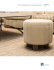### Lucy Lu | Lounge Seating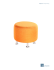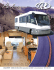### BaBy Spinach Salad with champagne Vinaigrette and maple glazed pecanS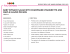### Click here - New Britain Youth Museum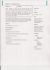### Golden Full Moon Maple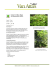### plant introductions - Jeffries Nurseries Ltd.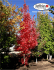### Seiryu Japanese Maple